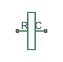# Translational Inerter

Two-port inertia in mechanical translational systems

## Library

Mechanical Translational Elements

•## Description

The Translational Inerter block represents a device that has force proportional to the rate of change of the relative velocity across the ports. It is essentially a two-port inertia that works on the velocity difference between the ports, not the absolute velocity. An inerter is the mechanical equivalent of a capacitor. An inerter with one port connected to ground essentially behaves as a mass with the mass equal to the inerter’s inertance.

Use this block in high performance suspension systems, to decouple weave and roll modes, or in applications where you need to model a passively tuned mass-spring-damper response.

The block is described with the following equations:

`$f=B\frac{dv}{dt}$`
`$v={v}_{R}-{v}_{C}$`

where

 F Force transmitted through the inerter B Inertance v Relative velocity vR, vC Absolute velocities at ports R and C, respectively

The block positive direction is from port R to port C. This means that the force is positive if it acts in the direction from R to C.

### Variables

To set the priority and initial target values for the block variables prior to simulation, use the Initial Targets section in the block dialog box or Property Inspector. For more information, see Set Priority and Initial Target for Block Variables.

Nominal values provide a way to specify the expected magnitude of a variable in a model. Using system scaling based on nominal values increases the simulation robustness. Nominal values can come from different sources, one of which is the Nominal Values section in the block dialog box or Property Inspector. For more information, see Modify Nominal Values for a Block Variable.

## Parameters

Inertance

Proportionality coefficient between the force and the rate of change of the relative velocity across the ports. The default value is `1` kg.

## Ports

The block has the following ports:

`R`

Mechanical translational conserving port associated with the rod.

`C`

Mechanical translational conserving port associated with the case.

## Version History

Introduced in R2015b